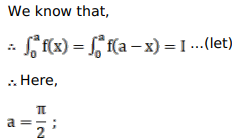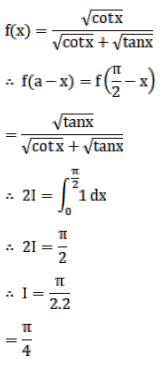# Mark against the correct answer in the following:

Question:

Mark $(\sqrt{ })$ against the correct answer in the following:

$\int_{0}^{\pi / 2} \frac{\sqrt{\cot x}}{\sqrt{\cot x}+\sqrt{\tan x}} d x=?$

A. 0

B. $\frac{\pi}{2}$

C. $\frac{\pi}{4}$

D. none of these

Solution: